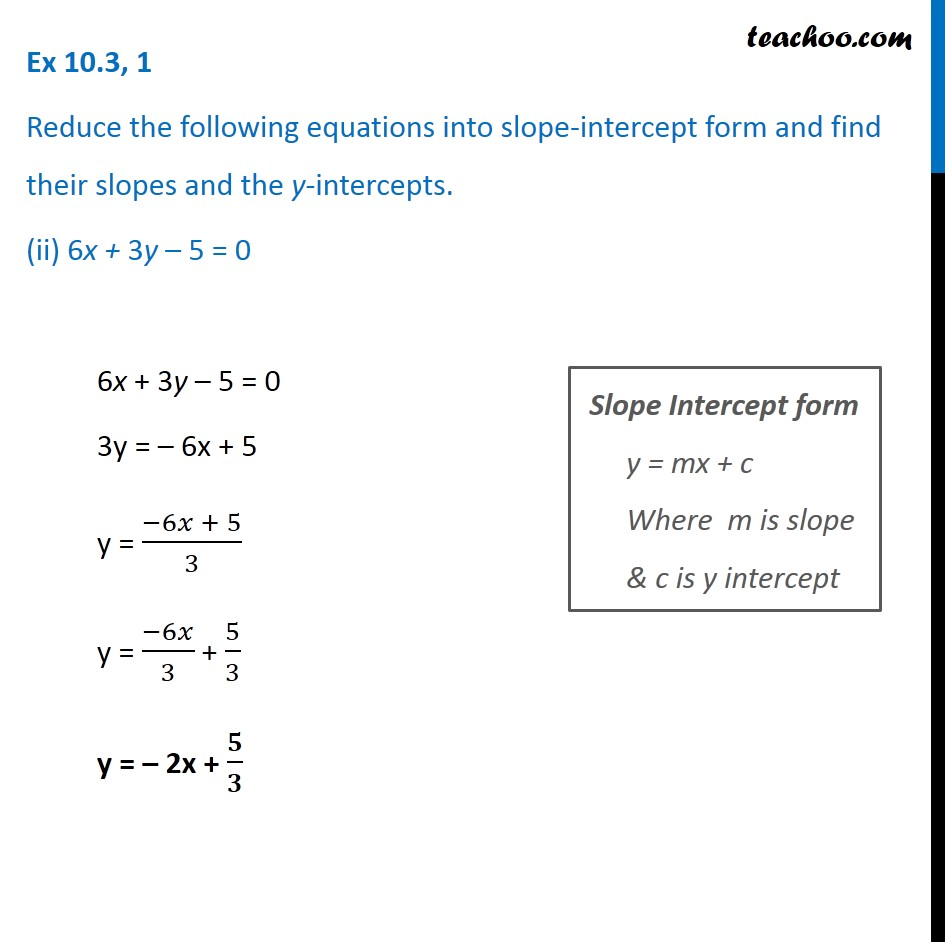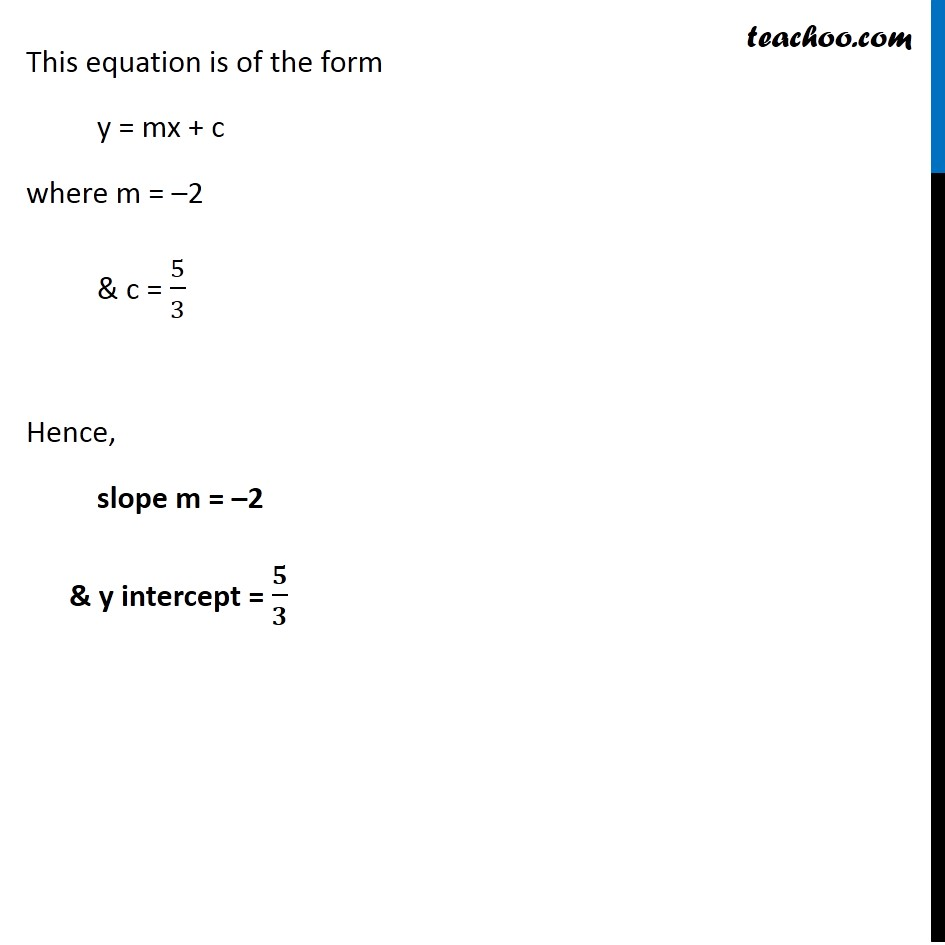Ex 9.3

Chapter 9 Class 11 Straight Lines
Serial order wiseLearn in your speed, with individual attention - Teachoo Maths 1-on-1 Class

### Transcript

Ex 9.3, 1 Reduce the following equations into slope-intercept form and find their slopes and the y-intercepts. (ii) 6x + 3y – 5 = 0 6x + 3y – 5 = 0 3y = – 6x + 5 y = (−6𝑥 + 5)/3 y = (−6𝑥)/3 + 5/3 y = – 2x + 𝟓/𝟑 Slope Intercept form y = mx + c Where m is slope & c is y intercept This equation is of the form y = mx + c where m = –2 & c = 5/3 Hence, slope m = –2 & y intercept = 𝟓/𝟑